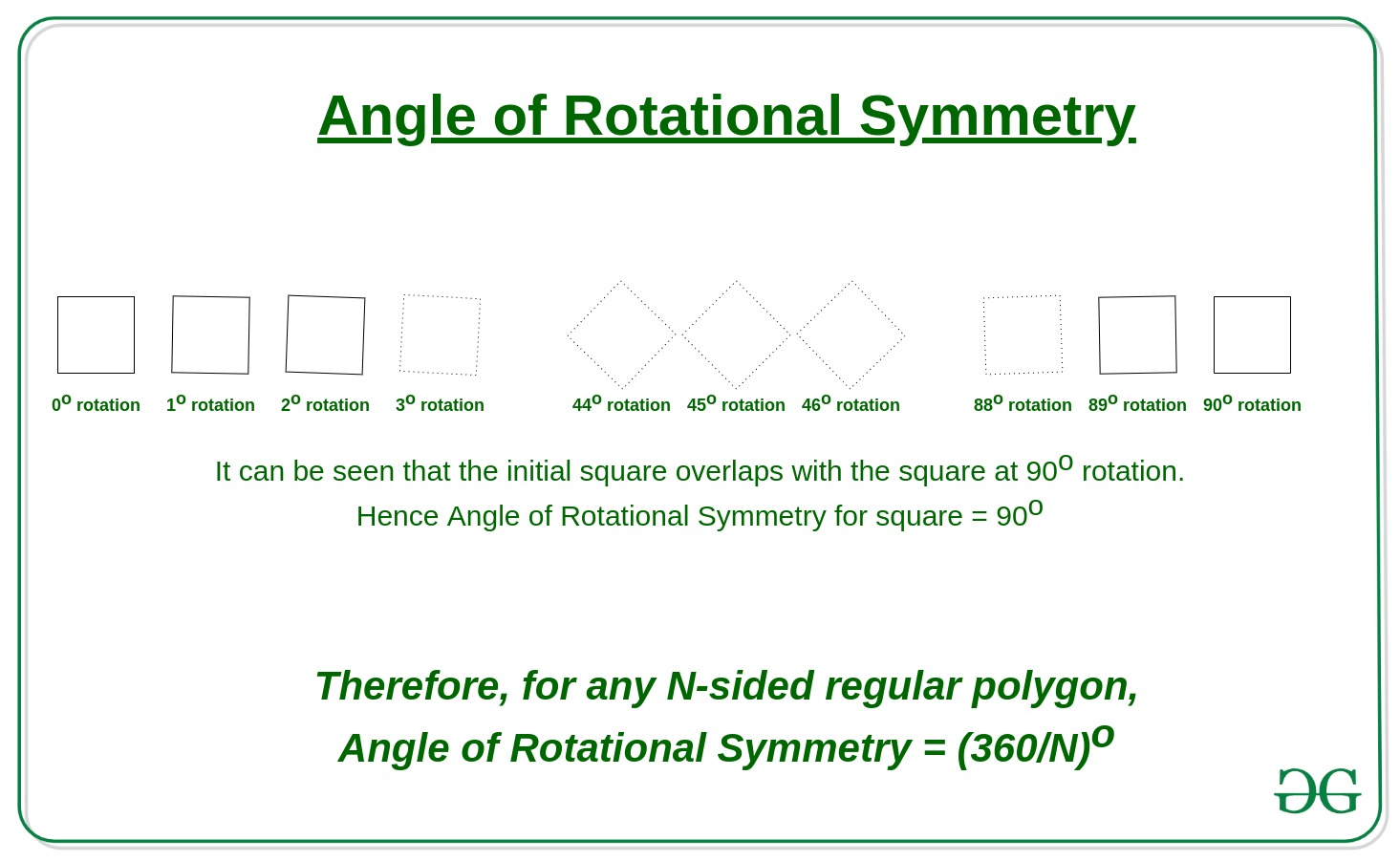# Find the angle of Rotational Symmetry of an N-sided regular polygon

Given an integer N which is the number of sides of a regular polygon. The task is to find the smallest angle of rotation such that the generated regular polygons have a similar position and dimensions, i.e. the new rotated polygon is in symmetry with the initial one.

A shape is said to have a rotation symmetry if there exists a rotation in the range [1, 360o] such that the new shape overlaps the initial shape completely.

Examples:

Input: N = 4
Output: 90
Explanation:
A 4 sided regular polygon is a square and when it is rotated by 90 degrees it results in the similar square.
Input: N = 8
Output: 45

Approach: For any N sided regular polygon, when rotated by 360 degrees, it aligns in the original position of the polygon. To find the minimum angle of rotation we use the property of symmetry of regular polygons. For an N sided regular polygon when rotated by 360/N degrees, the rotated polygon is in the same position as of the original polygon, which is the exterior angle of an N-sided regular polygon.
For example:
Consider N = 4,Below is the implementation of the above approach.

## C++

 `// C++ program to find the angle` `// of Rotational Symmetry of` `// an N-sided regular polygon`   `#include ` `using` `namespace` `std;`   `// function to find required` `// minimum angle of rotation` `double` `minAnglRot(``int` `N)` `{` `    ``// Store the answer in` `    ``// a double variable` `    ``double` `res;`   `    ``// Calculating the angle` `    ``// of rotation and type-` `    ``// casting the integer N` `    ``// to double type` `    ``res = 360 / (``double``)N;`   `    ``return` `res;` `}`   `// Driver code` `int` `main()` `{` `    ``int` `N = 4;` `    ``cout << ``"Angle of Rotational Symmetry: "` `         ``<< minAnglRot(N);` `    ``return` `0;` `}`

## Java

 `// Java program to find the angle` `// of Rotational Symmetry of` `// an N-sided regular polygon` `import` `java.io.*;` `class` `GFG ` `{`   `// function to find required` `// minimum angle of rotation` `static` `double` `minAnglRot(``int` `N)` `{` `    ``// Store the answer in` `    ``// a double variable` `    ``double` `res;`   `    ``// Calculating the angle` `    ``// of rotation and type-` `    ``// casting the integer N` `    ``// to double type` `    ``res = ``360` `/ (``double``)N;`   `    ``return` `res;` `}`   `// Driver code` `public` `static` `void` `main (String[] args) ` `{` `    ``int` `N = ``4``;` `    ``System.out.println(``"Angle of Rotational Symmetry: "` `+ ` `                                          ``minAnglRot(N));` `}` `}`   `// This code is contributed by shivanisinghss2110`

## Python3

 `# Python3 program to find the angle` `# of Rotational Symmetry of` `# an N-sided regular polygon`   `# Function to find required` `# minimum angle of rotation` `def` `minAnglRot(N):` `    `  `    ``# Store the answer in a ` `    ``# variable` `    `  `    ``# Calculating the angle` `    ``# of rotation and type-` `    ``# casting the integer N` `    ``# to type` `    ``res ``=` `360` `/``/` `N`   `    ``return` `res`   `# Driver code` `if` `__name__ ``=``=` `'__main__'``:` `    `  `    ``N ``=` `4``;` `    `  `    ``print``(``"Angle of Rotational Symmetry: "``, ` `          ``minAnglRot(N))`   `# This code is contributed by mohit kumar 29    `

## C#

 `// C# program to find the angle` `// of Rotational Symmetry of` `// an N-sided regular polygon` `using` `System;` `class` `GFG ` `{` ` `  `// function to find required` `// minimum angle of rotation` `static` `double` `minAnglRot(``int` `N)` `{` `    ``// Store the answer in` `    ``// a double variable` `    ``double` `res;` ` `  `    ``// Calculating the angle` `    ``// of rotation and type-` `    ``// casting the integer N` `    ``// to double type` `    ``res = 360 / (``double``)N;` ` `  `    ``return` `res;` `}` ` `  `// Driver code` `public` `static` `void` `Main (``string``[] args) ` `{` `    ``int` `N = 4;` `    ``Console.Write(``"Angle of Rotational Symmetry: "` `+ ` `                                     ``minAnglRot(N));` `}` `}` ` `  `// This code is contributed by rock_cool`

Output:

```Angle of Rotational Symmetry: 90

```

Time Complexity: O (1)
Auxiliary Space: O (1)

Attention reader! Don’t stop learning now. Get hold of all the important DSA concepts with the DSA Self Paced Course at a student-friendly price and become industry ready.

My Personal Notes arrow_drop_upCheck out this Author's contributed articles.

If you like GeeksforGeeks and would like to contribute, you can also write an article using contribute.geeksforgeeks.org or mail your article to contribute@geeksforgeeks.org. See your article appearing on the GeeksforGeeks main page and help other Geeks.

Please Improve this article if you find anything incorrect by clicking on the "Improve Article" button below.